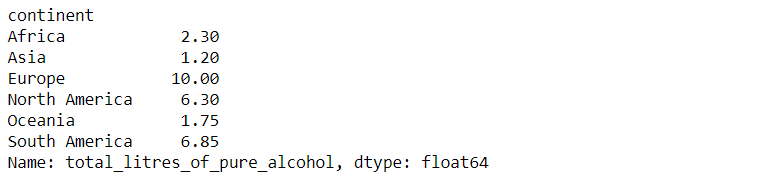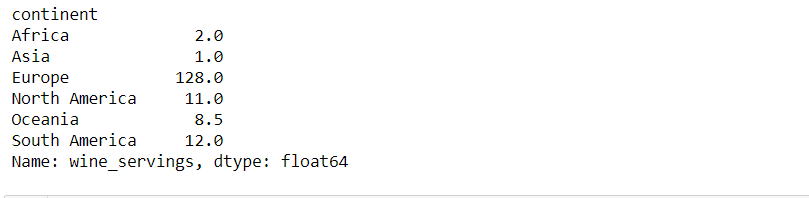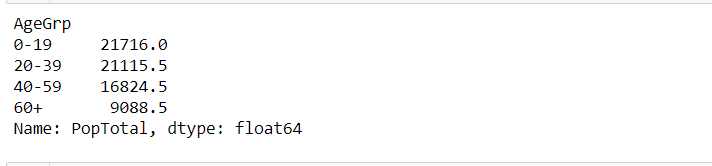GFG App
Open AppBrowser
Continue

The Pandas in Python is known as the most popular and powerful tool for performing data analysis. It because of the beauty of Pandas functionality and the ability to work on sets and subsets of the large dataset. So in this article, we are going to study how pandas Group By functionality works and saves tons of effort while working on a large dataset. Also, we will solve real-world problems using Pandas Group By and Median functionalities.

### Pandas groupby()

The groupby() method in pandas splits the dataset into subsets to make computations easier. Generally, groupby() splits the data, applies the functionalities, and then combine the result for us. Let’s take an example if we have data on alcohol consumption of different countries and we want to perform data analysis continent-wise, this problem can be minimized using groupby() method in pandas. It splits the data continent-wise and calculates median using the median() method.

Syntax :

DataFrame.groupby(by=None, axis=0, level=None, as_index=True, sort=True, group_keys=True, squeeze=<object object>, observed=False, dropna=True)

Example 1: Find the median of alcohol consumption continent-wise on a given dataset.

Dataset: Drinksbycountry.csv

## Python3

 `# import the packages` `import` `pandas as pd`   `# read Dataset` `data ``=` `pd.read_csv(``"drinksbycountry.csv"``)` `data.head()`   `# perform groupby on continent and find median` `# of total_litres_of_pure_alcohol` `data.groupby([``"continent"``])[``"total_litres_of_pure_alcohol"``].median()`   `# perform groupby on continent and find median ` `# of wine_serving` `data.groupby([``"continent"``])[``"wine_servings"``].median()`

Output :median of total_litres_of_pure_alcoholmedian of wine_serving

Example 2: Find the median of the total population group by age on a given dataset.

Dataset: WorldPopulationByAge2020.csv

## Python3

 `# import packages` `import` `pandas as pd`   `# read Dataset` `data ``=` `pd.read_csv(``"WorldPopulationByAge2020.csv"``)` `data.head()`   `# perform group by AgeGrp and find median` `data.groupby([``"AgeGrp"``])[``"PopTotal"``].median()`

Output :Group by Age

My Personal Notes arrow_drop_up